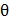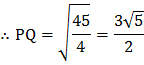# A curve is given by the equations. If the tangent atwheremeets the curve again at, thenis, whererepresents the greatest integer function

## Question ID - 153414 :- A curve is given by the equations. If the tangent atwheremeets the curve again at, thenis, whererepresents the greatest integer function

3537

 (3)atAlso the pointforis (2, 1) Equation of tangent isor(1) This meets the curve whose Cartesian equation on eliminatingbyis(2) Solving (1) and (2), we getHenceis (2,1) as given andisFrom a given solid cone of height, another inverted cone is carved whose height issuch that its volume is maximum. Then the ratiois